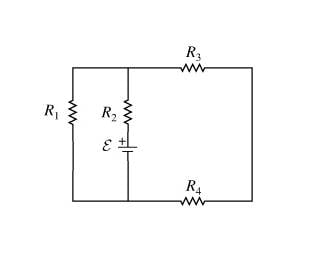# Current through each individual resistor of a circuit

Linus Pauling
1.What is the current through each resistor

2. All the obvious stuff...

3. Ok, without showing all my work, here's what I have solved and know is right:

The equivalent resistance of the whole circuit is 56.3 ohm. From this, I used Ohm's law to get the equivalent current for the circuit, which is 0.213A.

Where I am stuck is now using this to get the individual currents for each resistor. For example, I calculated I_2 = V/R_2 = 12V/45ohm = 0.27A

Similarly, I found found the others, so here's what I got (which is incorrect):

I_1 = 0.8A
I_2 = 0.27
I_3 = 0.48
I_4 = 0.6

Also, I notice that these values sum to 2.12, which approximately euqals 2.13, which is the equivalent current with the decimal point moved over.... where am I going wrong?

bigoldblack
It would help to show the values of each resistor and also showing the details for your current calculations.

Linus Pauling
Ahh, sorry for that:

V_bat = 12V
R1 = 15ohm
R2 = 45
R3 = 25
R4 = 20

My calculated values for the equivalent resistance and current of the circuit are correct. Briefly, R3 and 4 are in series, equiv resistor is in parallel with R1, and that equiv resistor is in series with R2.

Staff Emeritus